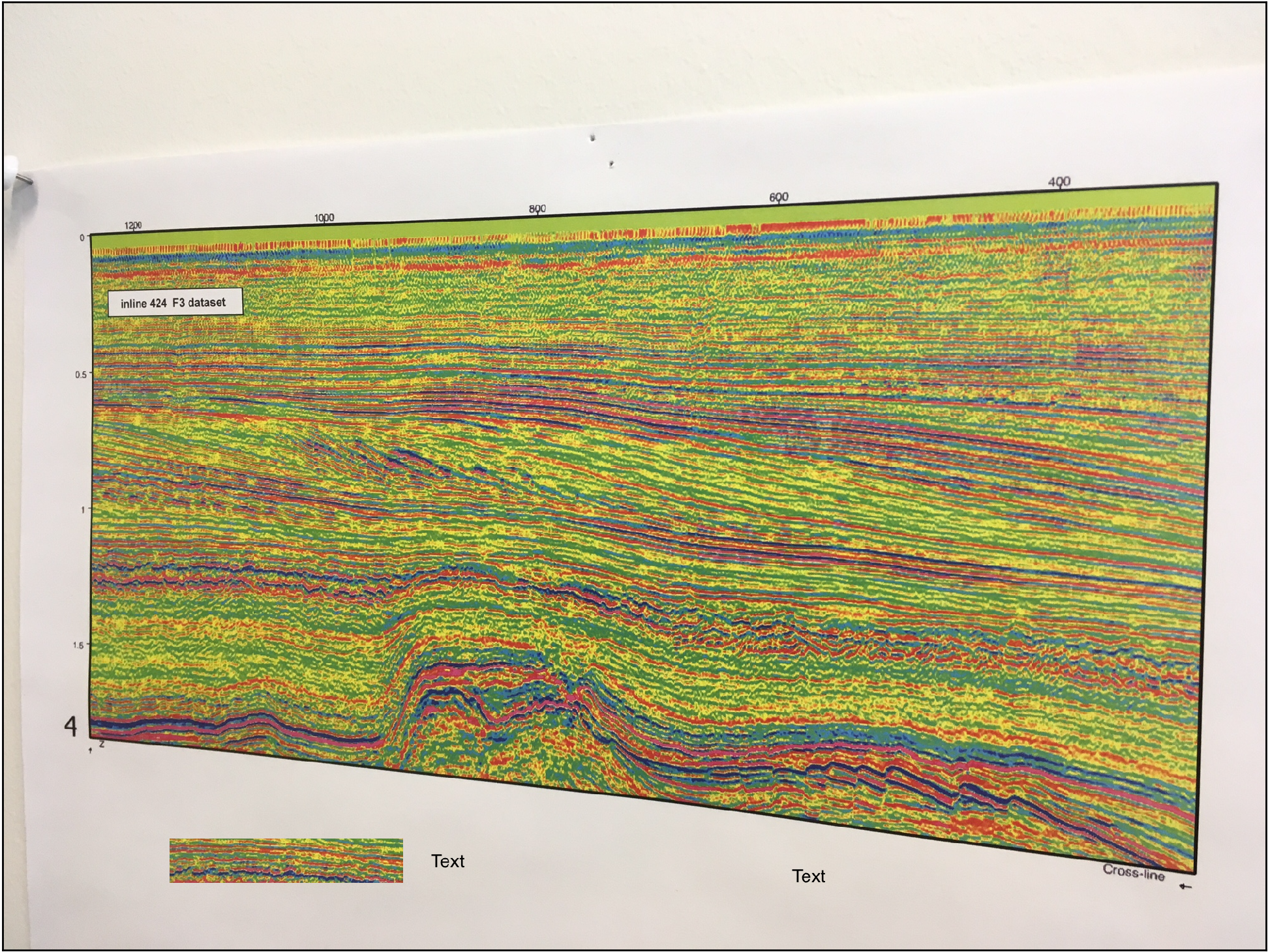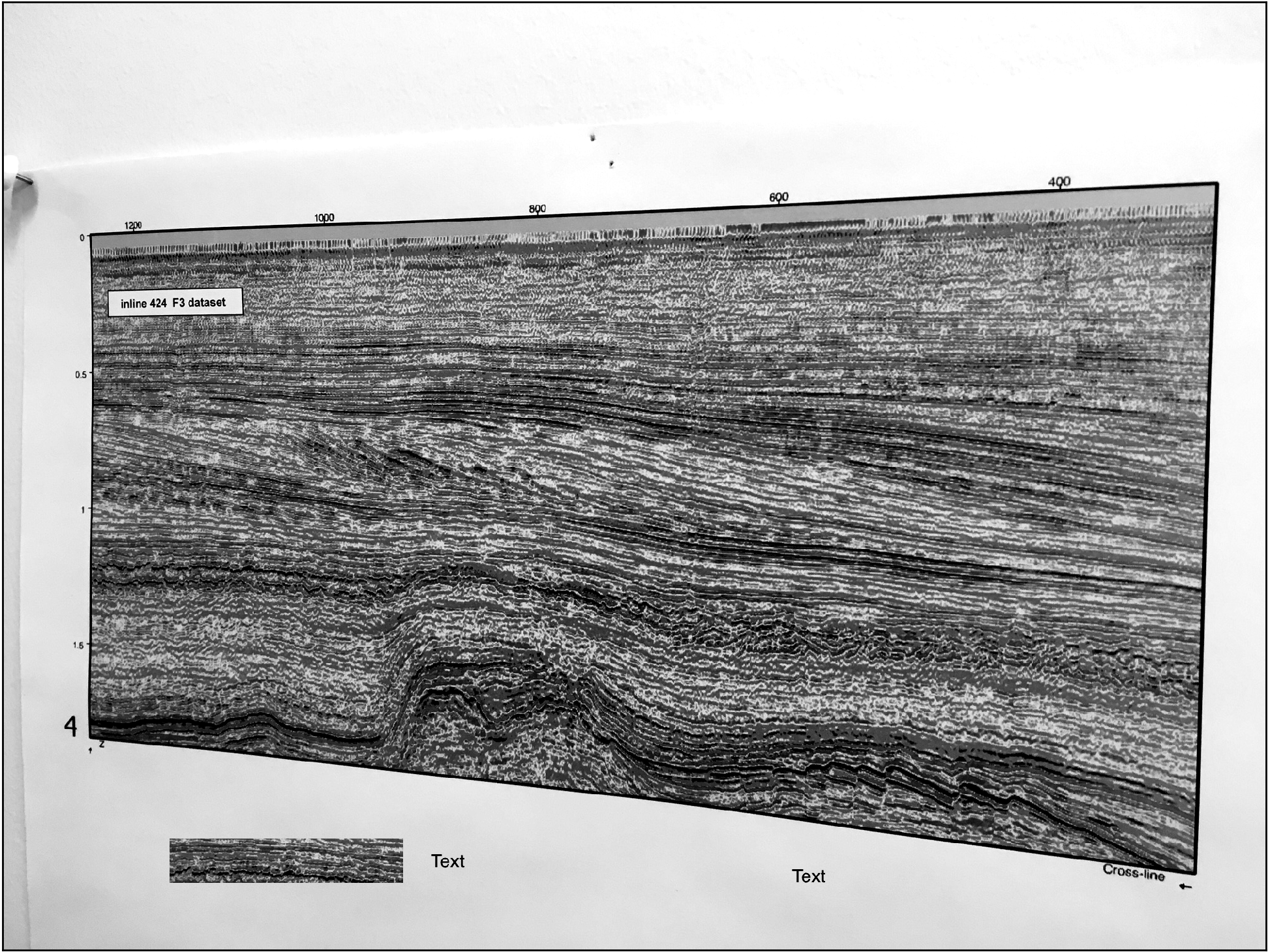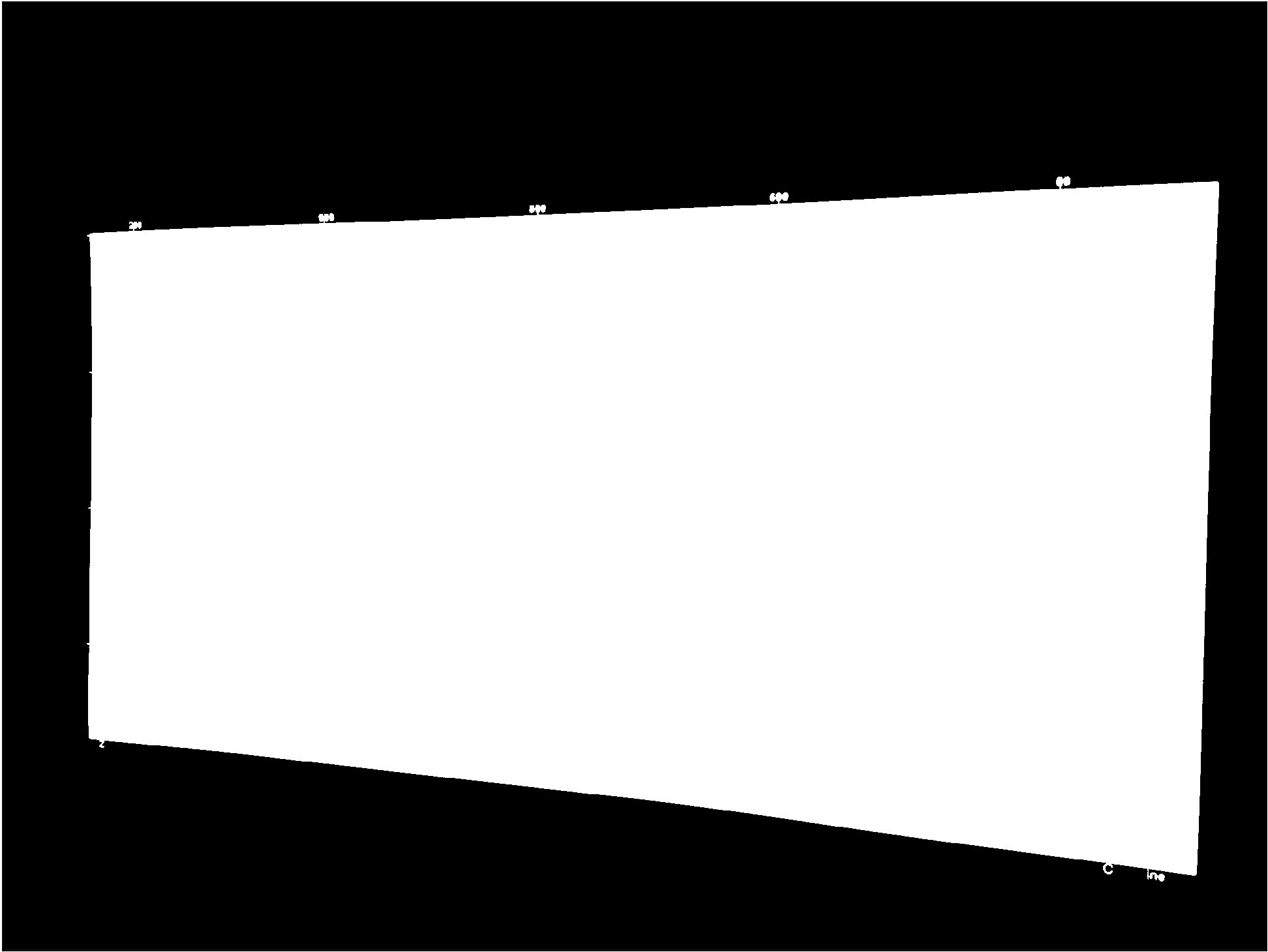# Computer vision in geoscience: recover seismic data from images – part 1

As anticipated in the introductory post of this short series I am going to demonstrate how to automatically detect where a seismic section is located in an image (be it a picture taken from your wall, or a screen capture from a research paper), rectify any distortions that might be present, and remove all sorts of annotations and trivia around and inside the section.

You can download from GitHub all the tools for the automated workflow (including both part 1 and part 2, and some of the optional features outlined in the introduction) in the module mycarta.py, as well as an example Jupyter Notebook showing how to run it.

In this part one I will be focusing on the image preparation and enhancement, and the automatic detection of the seismic section (all done using functions from numpy, scipy, and scikit-image)In order to do that, first I convert the input image  (Figure 1) containing the seismic section to grayscale and then enhance it by increasing the image contrast (Figure 2).Figure 1 – input imageFigure 2 – grayscale image

All it takes to do that is three lines of code as follows:

```gry = skimage.color.rgb2gray(img);
p2, p95 = numpy.percentile(gry, (2, 95))
rescale = exposure.rescale_intensity(gry, in_range=(p2, p95))```

For a good visual intuition of what actually is happening during the contrast stretching, check my post sketch2model – sketch image enhancements: in there  I show intensity profiles taken across the same image before and after the process.

Finding the seismic section in this image involve four steps:

1. converting the grayscale image to binary with a threshold (in this example a global threshold with the Otsu method)
2. finding and retaining only the largest object in the binary image (heuristically assumed to be the seismic section)
3. filling its holes
4. applying morphological operations to remove minutiae (tick marks and labels)

Below I list the code, and show the results.

```global_thresh = threshold_otsu(rescale)
binary_global = rescale < global_thresh```Figure 3 – binary image

```# (i) label all white objects (the ones in the binary image).
# scipy.ndimage.label actually labels 0s (the background) as 0 and then
# every non-connected, nonzero object as 1, 2, ... n.
label_objects, nb_labels = scipy.ndimage.label(binary_global)

# (ii) calculate every labeled object's binary size (including that
# of the background)
sizes = numpyp.bincount(label_objects.ravel())

# (3) set the size of the background to 0 so that if it happened to be
# larger than the largest white object it would not matter
sizes = 0

# (4) keep only the largest object
binary_objects = remove_small_objects(binary_global, max(sizes))

```Figure 4 – isolated seismic section

```# Remove holes (black regions inside white object)
binary_holes = scipy.ndimage.morphology.binary_fill_holes(binary_objects)```Figure 5 – holes removed

`enhanced = opening(binary_holes, disk(7))`Figure 6 – removed residual tick marks and labels

That’s it!!!

You can download from GitHub all the tools for the automated workflow (including both part 1 and part 2, and some of the optional features outlined in the introduction) in the module mycarta.py, as well as an example Jupyter Notebook showing how to run it.

In the next post, we will use this polygonal binary object both as a basis to capture the actual coloured seismic section from the input image and to derive a transformation to rectify it to a rectangle.